# RS Aggarwal Solutions for Class 12 Maths Chapter 10: Differentiation Exercise 10J

Second-order derivatives is the topic discussed under the Exercise 10J. Students who have doubts while solving the problems can refer to the PDF of solutions. The main aim is to improve logical thinking abilities and time management skills among students which are important from the exam point of view. Students can score good marks in the board exam by using RS Aggarwal Solutions Class 12 Maths Chapter 10 Differentiation Exercise 10J PDF as a vital study material during the exam preparation.

## RS Aggarwal Solutions for Class 12 Maths Chapter 10: Differentiation Exercise 10J Download PDF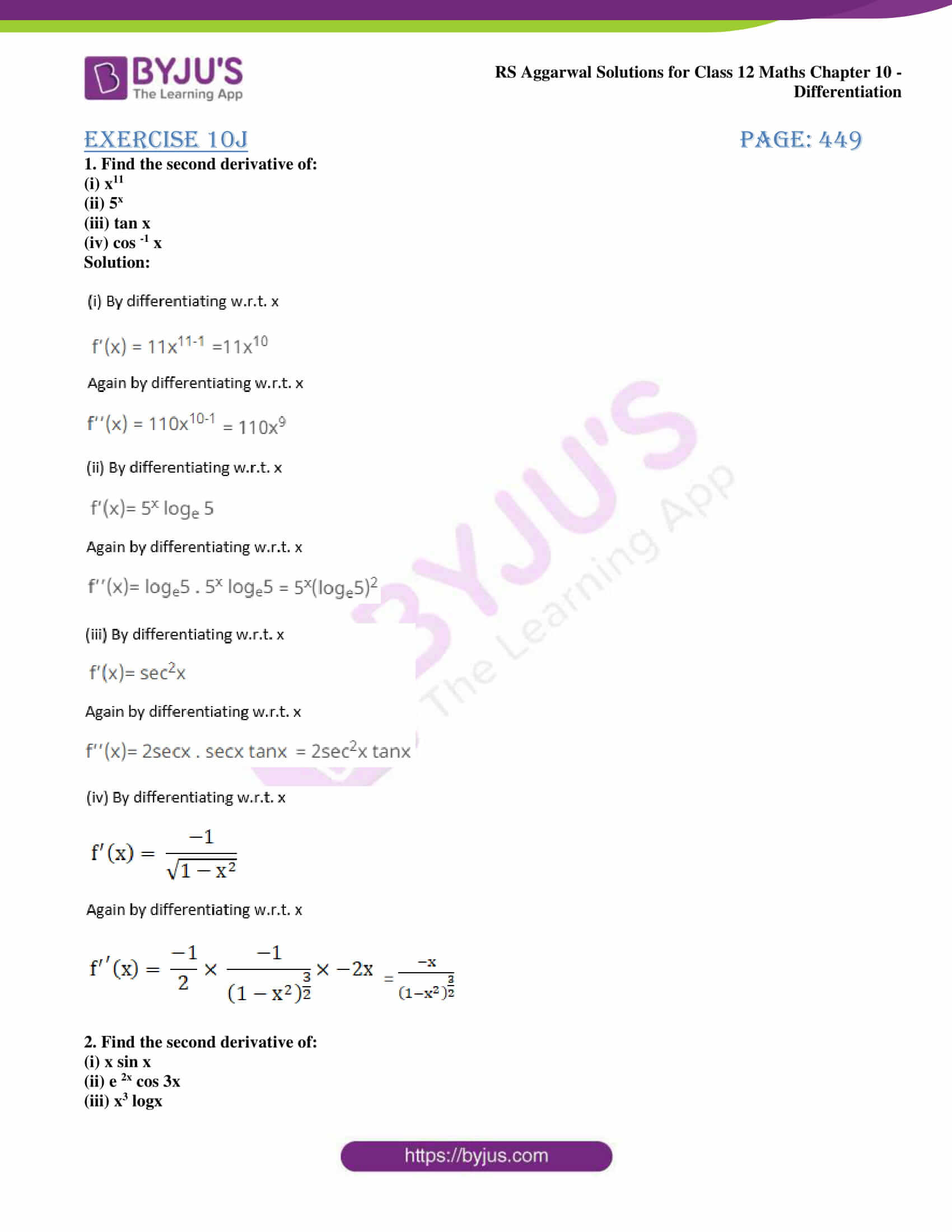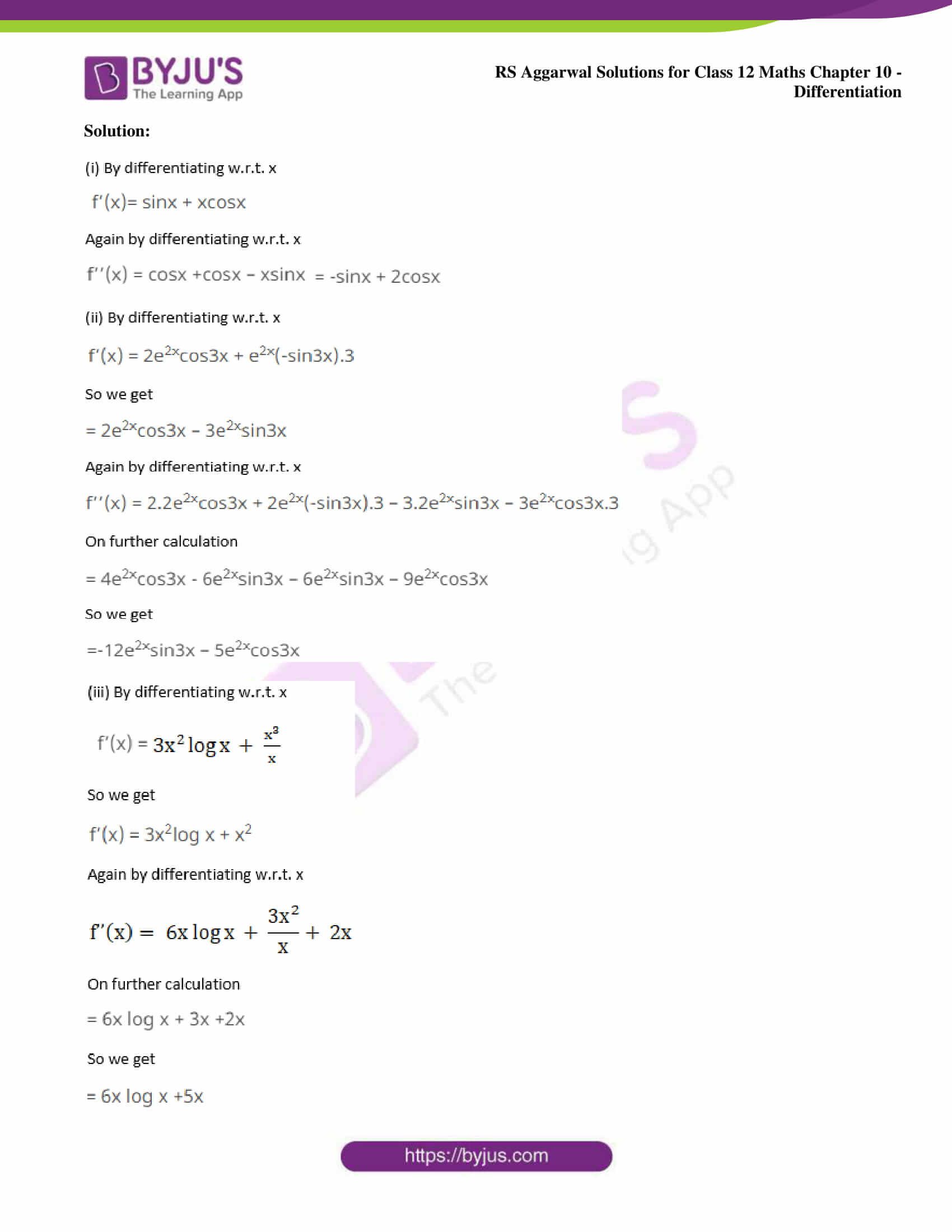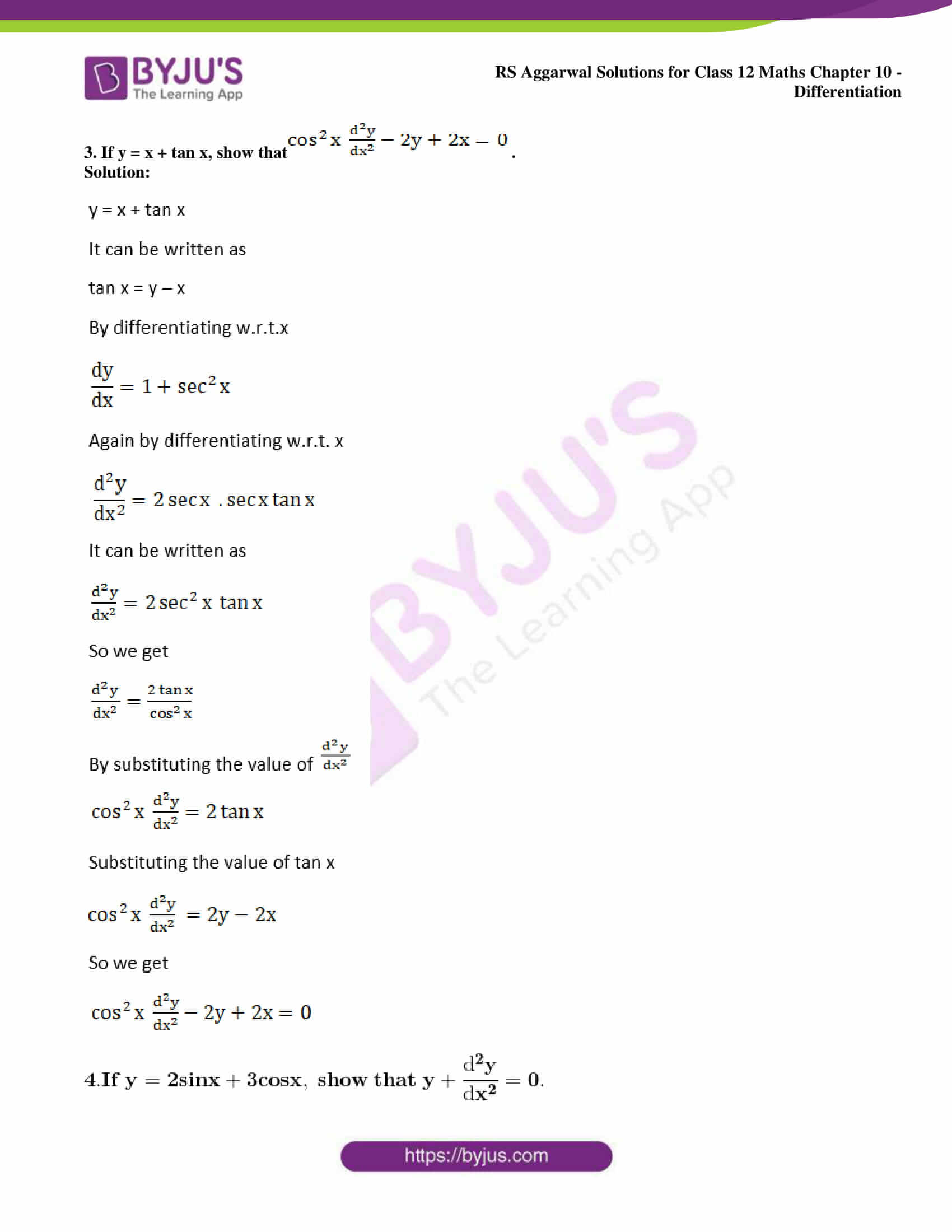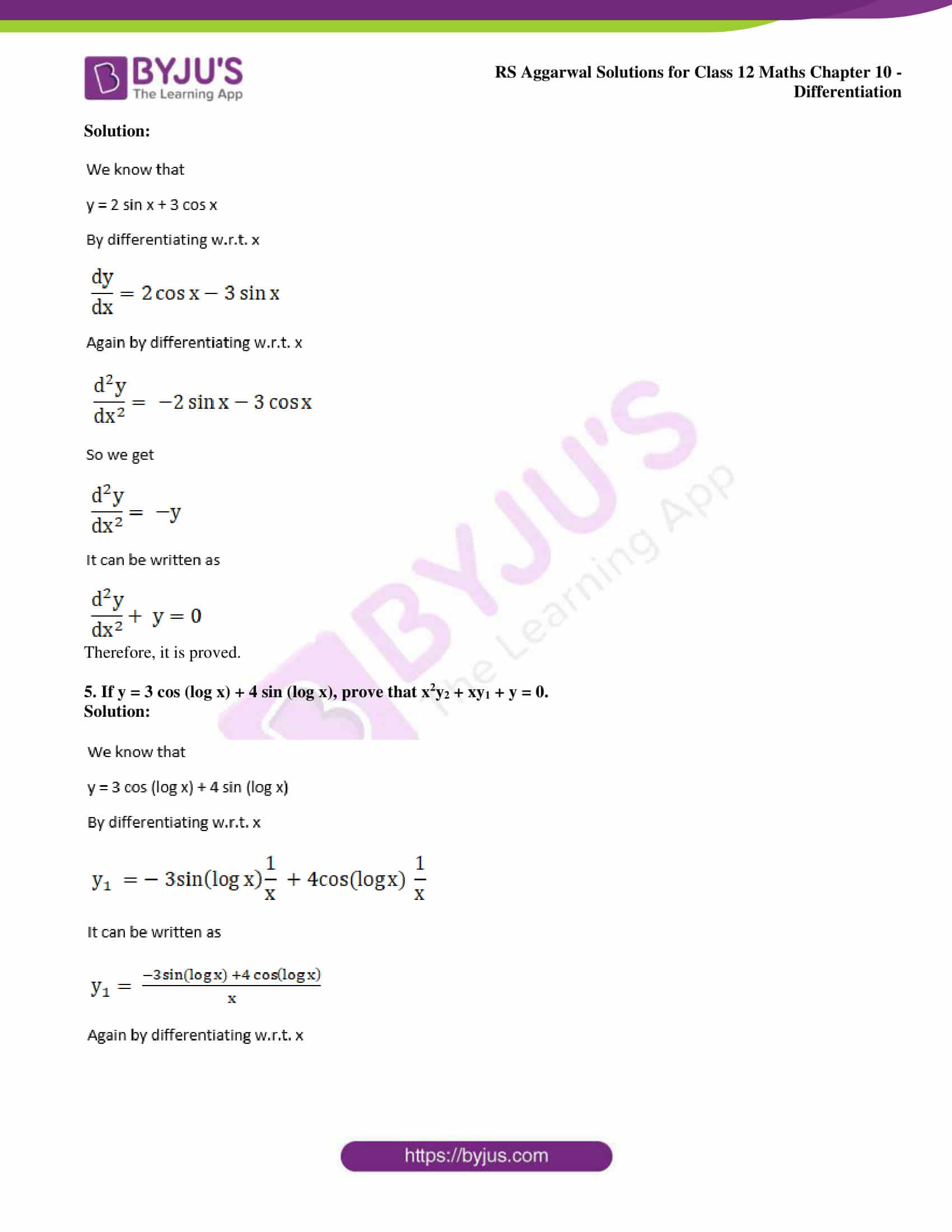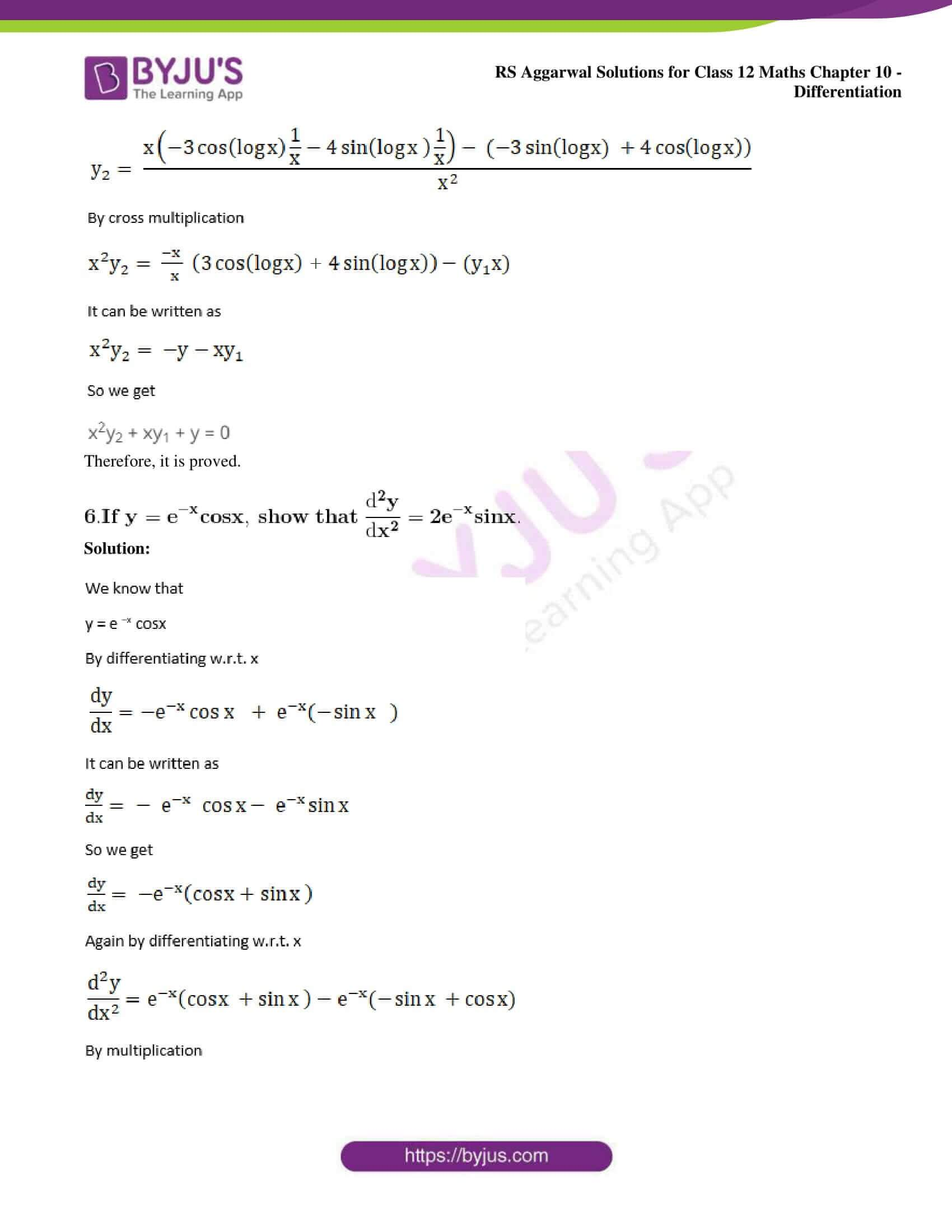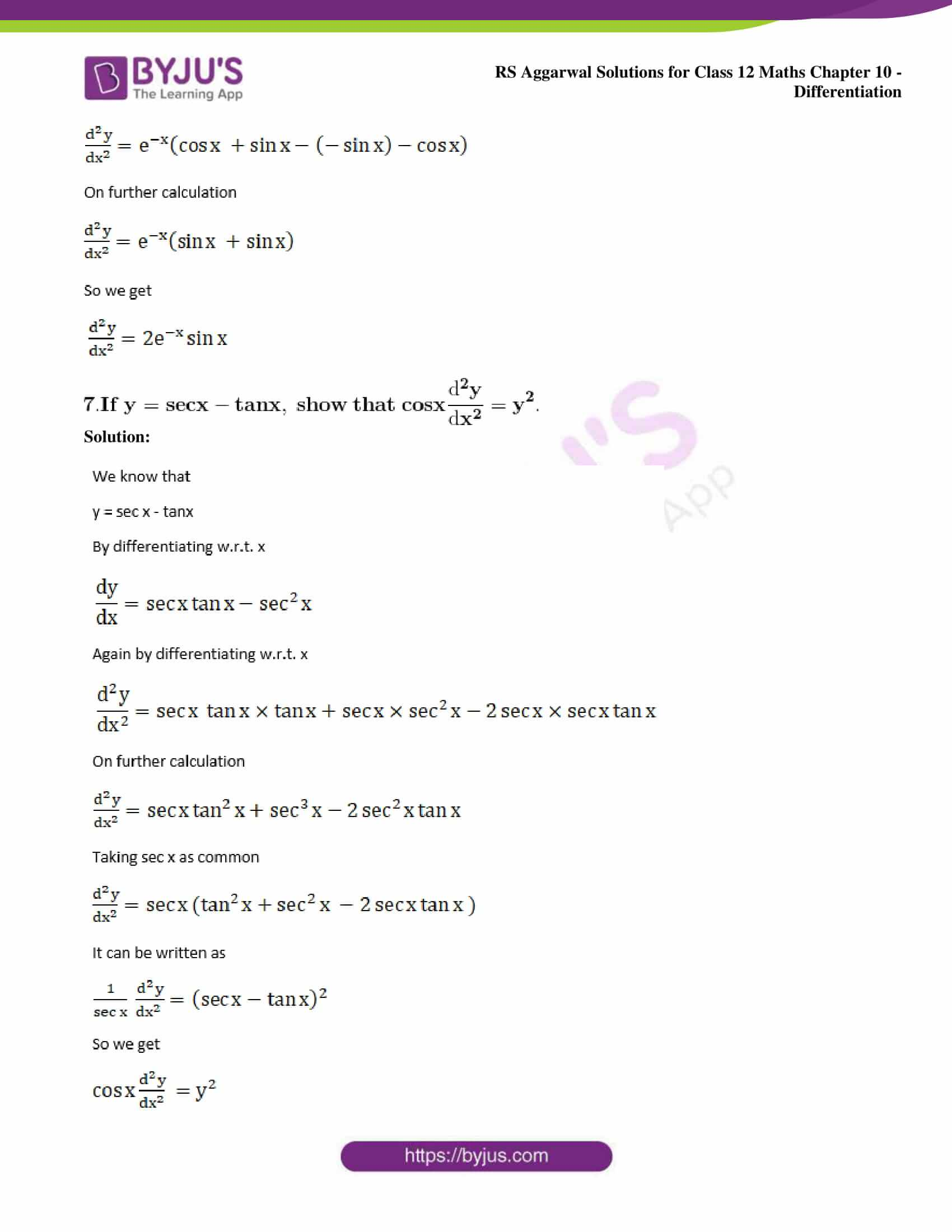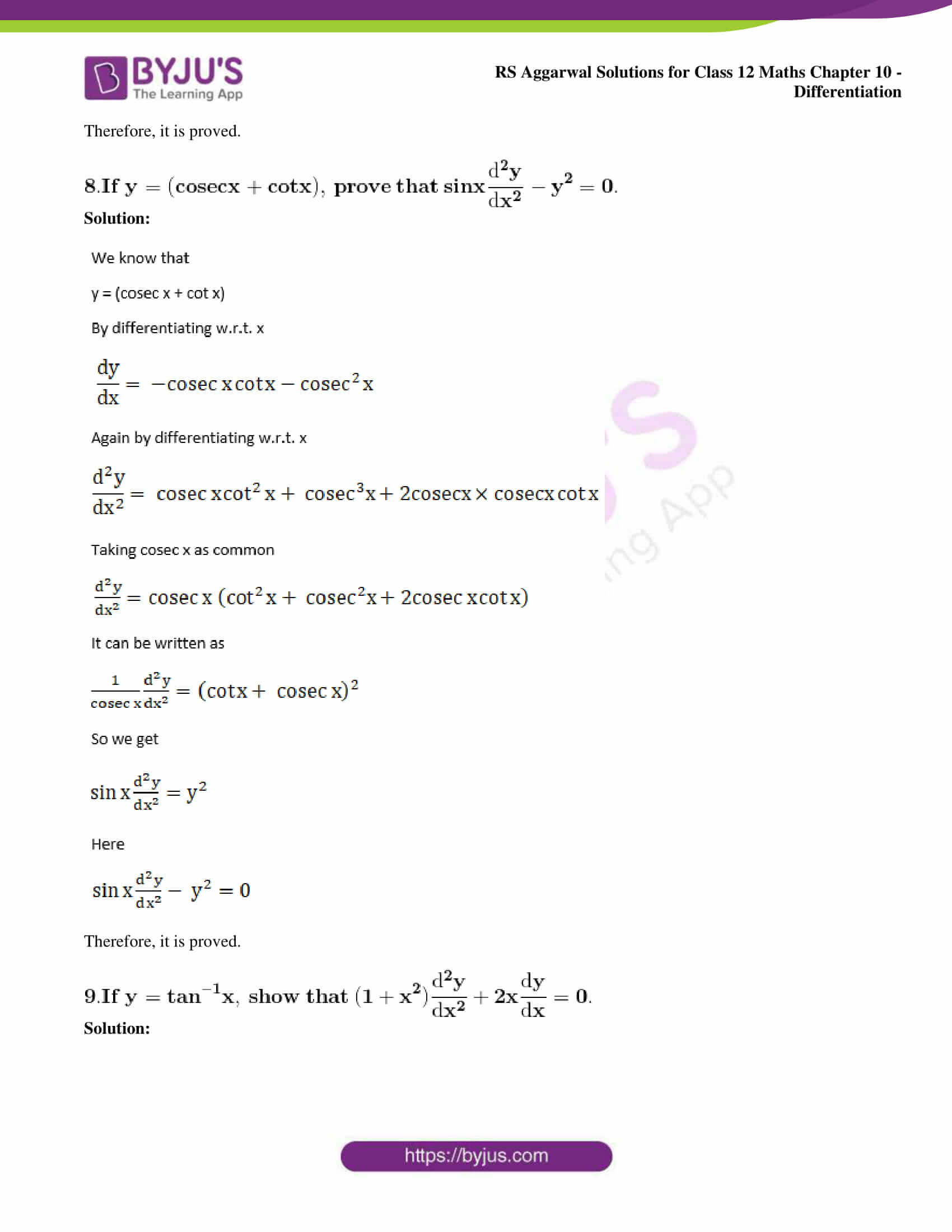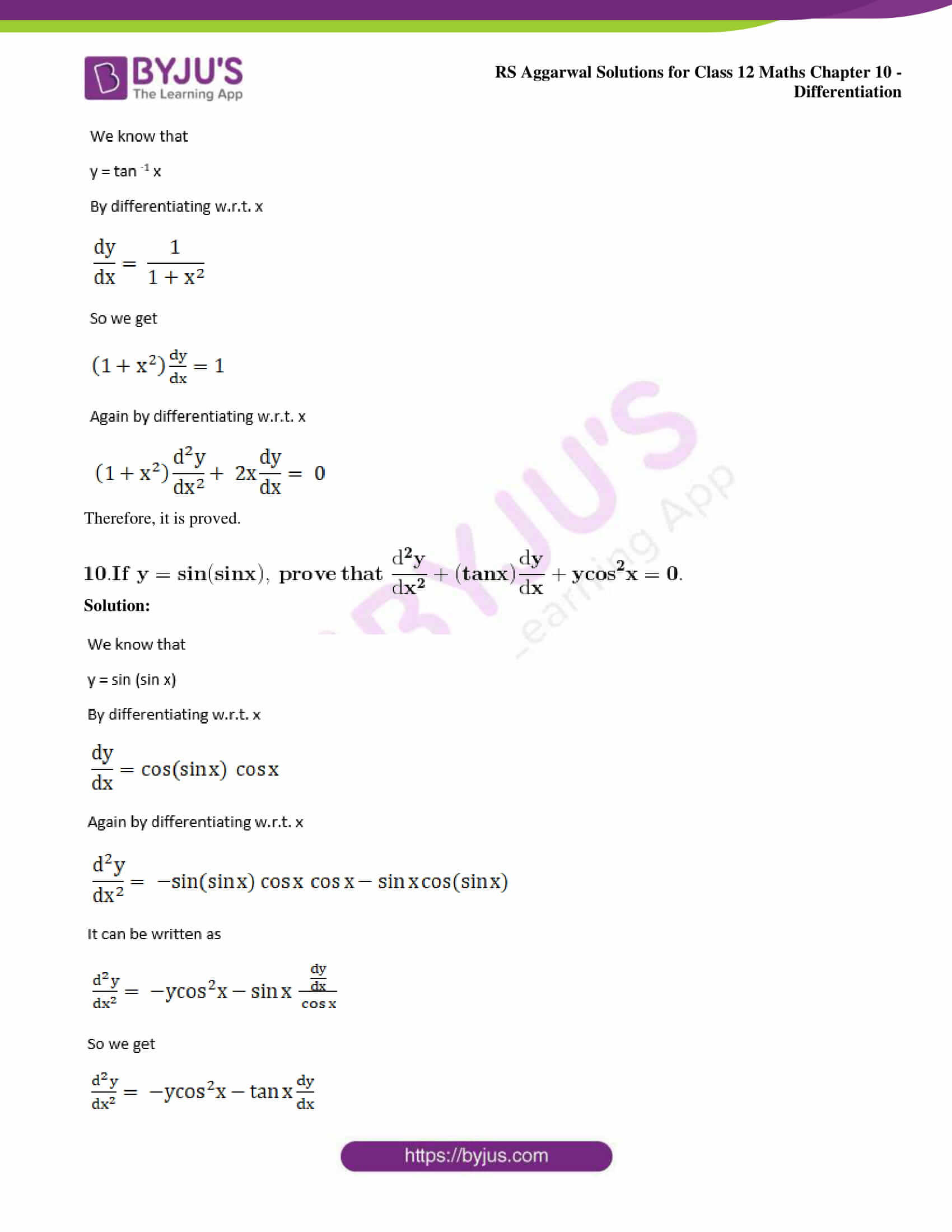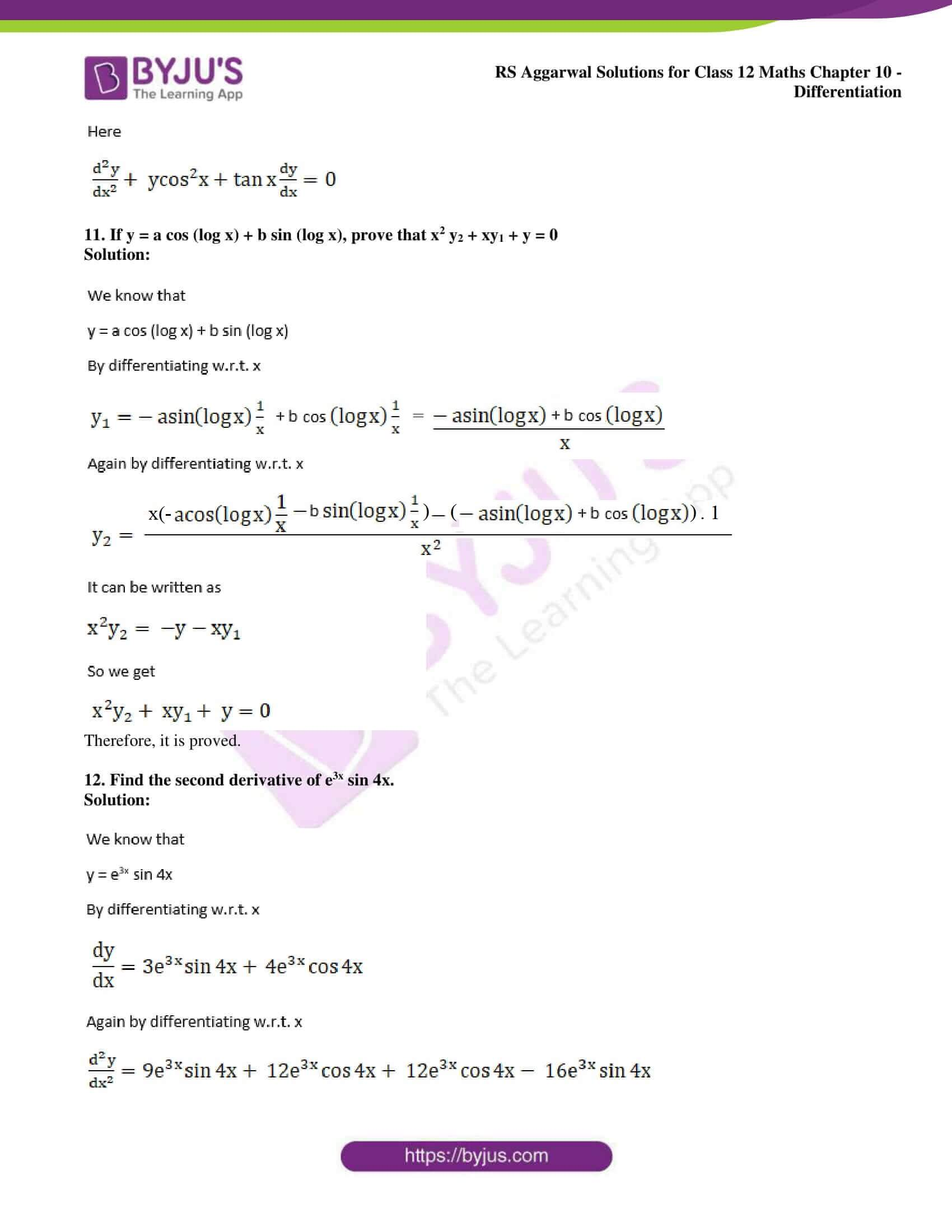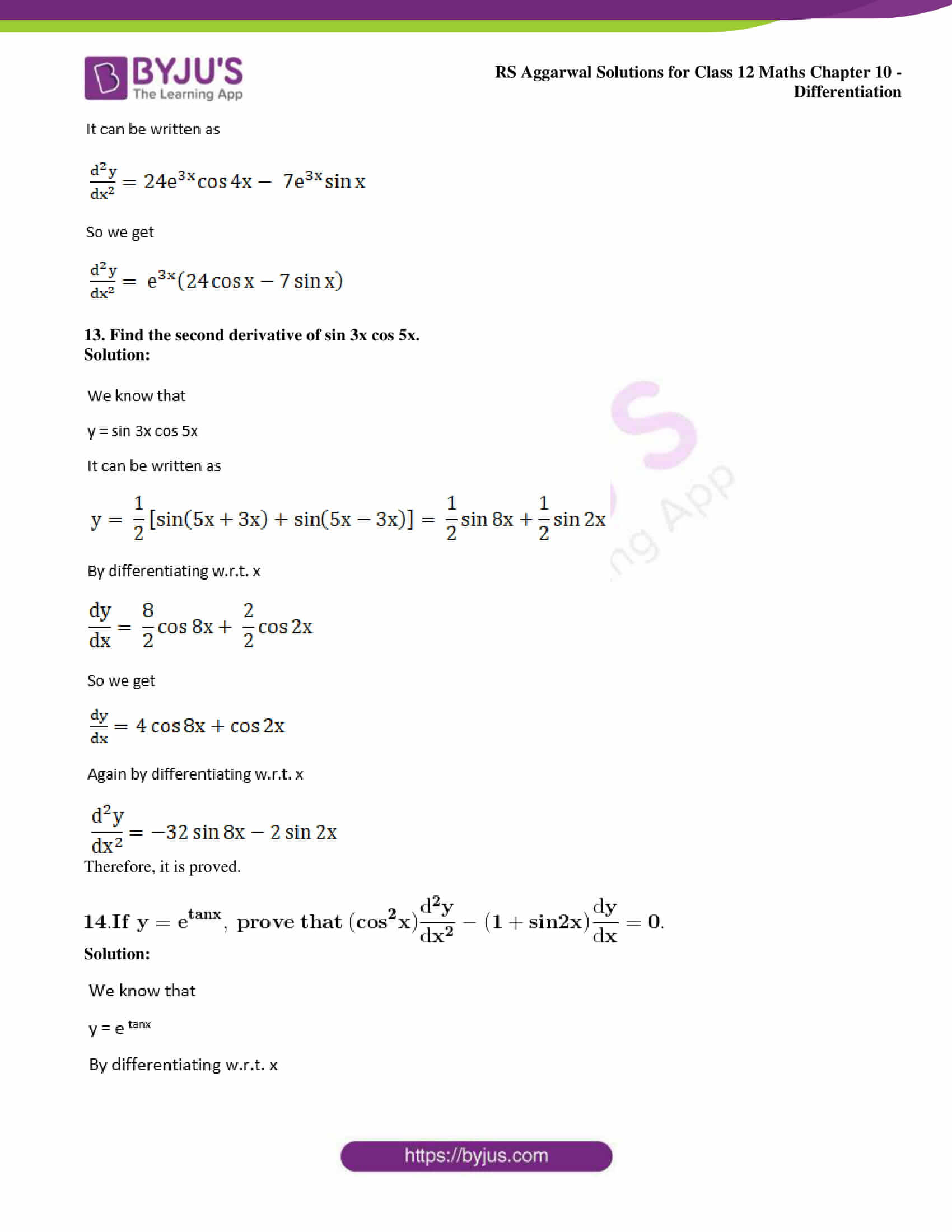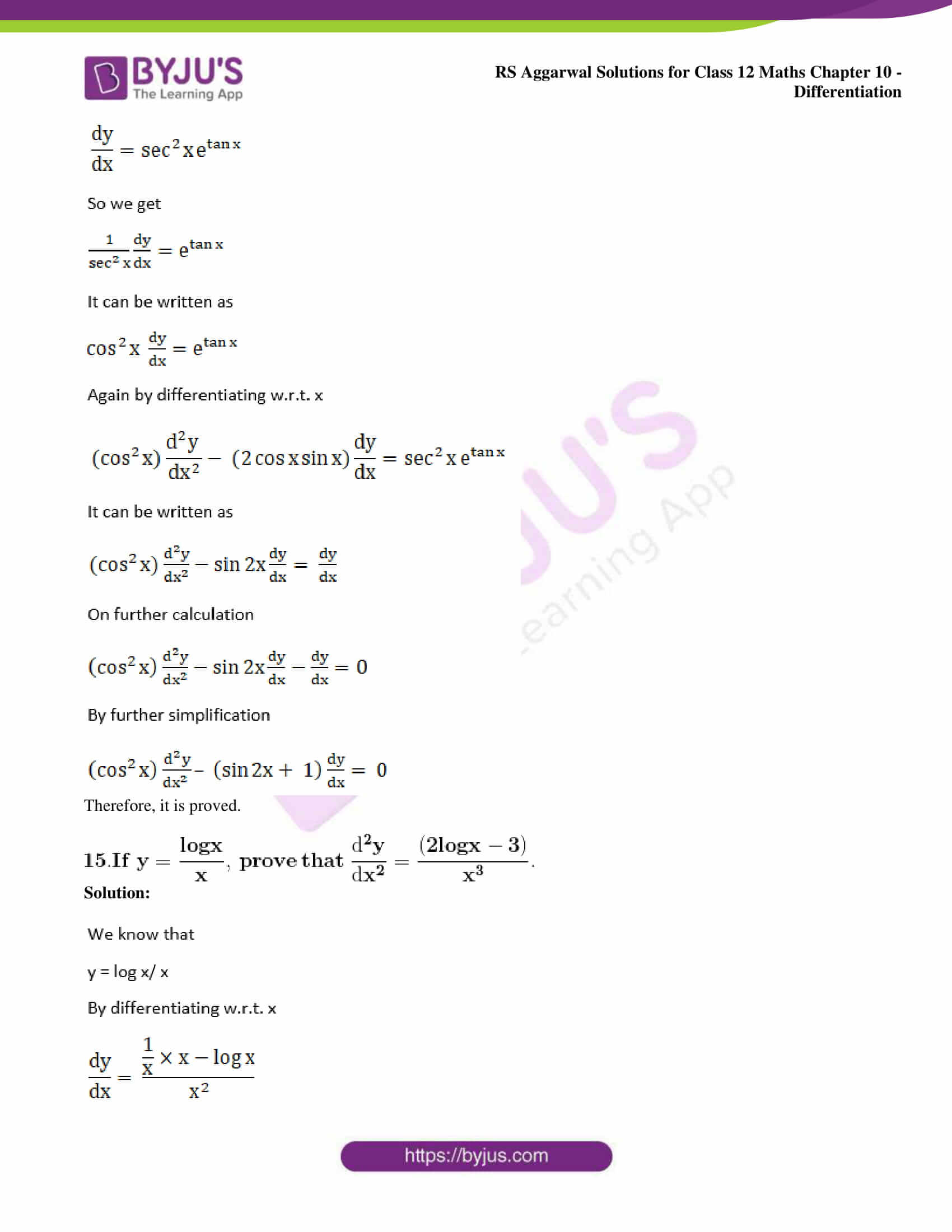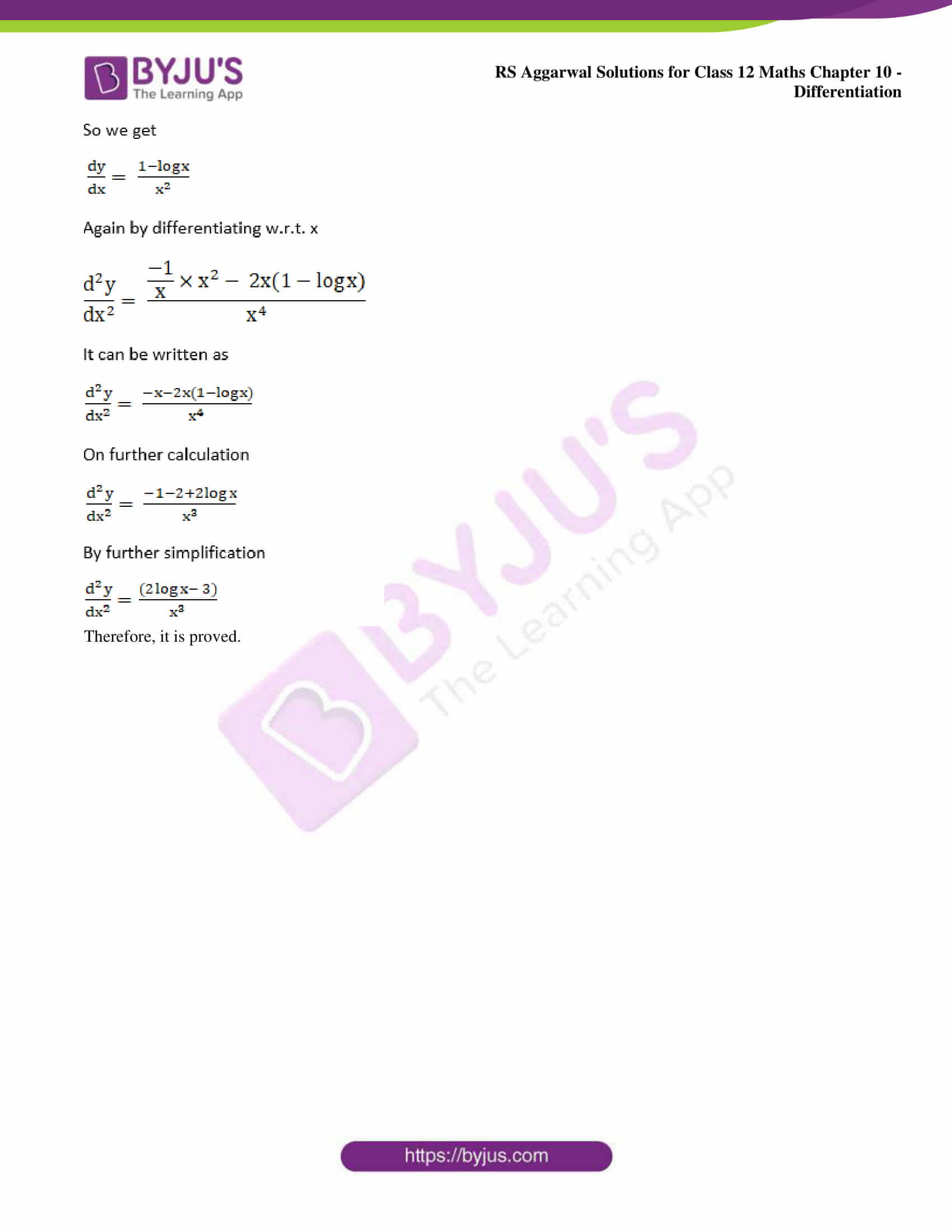### Access other exercise solutions of Class 12 Maths Chapter 10: Differentiation

Exercise 10A Solutions

Exercise 10B Solutions

Exercise 10C Solutions

Exercise 10D Solutions

Exercise 10E Solutions

Exercise 10F Solutions

Exercise 10G Solutions

Exercise 10H Solutions

Exercise 10I Solutions

### Access RS Aggarwal Solutions for Class 12 Maths Chapter 10: Differentiation Exercise 10J

1. Find the second derivative of:

(i) x11

(ii) 5x

(iii) tan x

(iv) cos -1 x

Solution: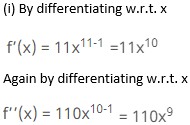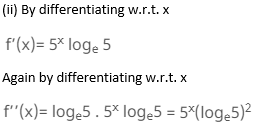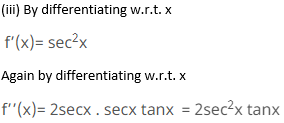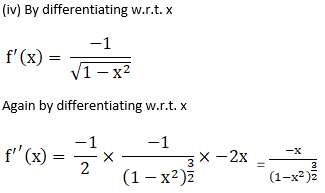2. Find the second derivative of:

(i) x sin x

(ii) e 2x cos 3x

(iii) x3 logx

Solution: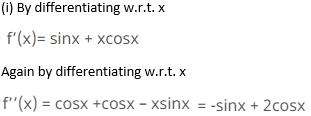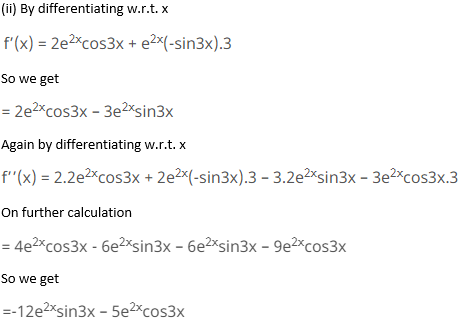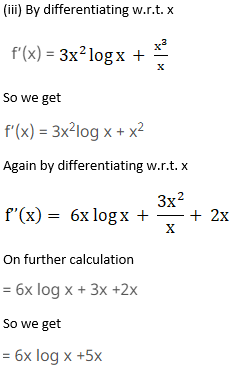3. If y = x + tan x, show that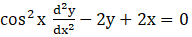.

Solution: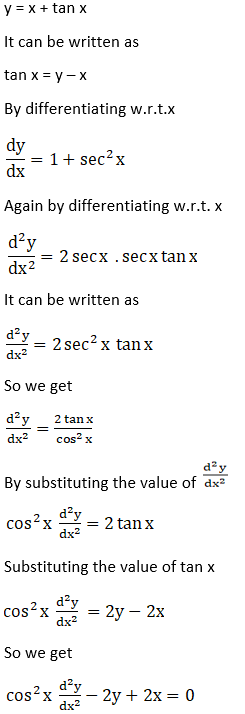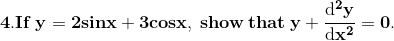Solution: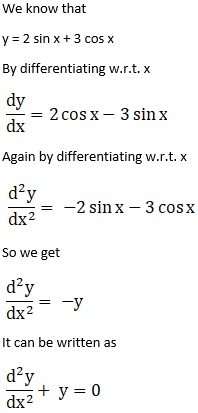Therefore, it is proved.

5. If y = 3 cos (log x) + 4 sin (log x), prove that x2y2 + xy1 + y = 0.

Solution: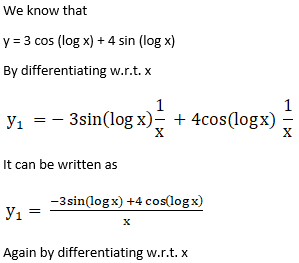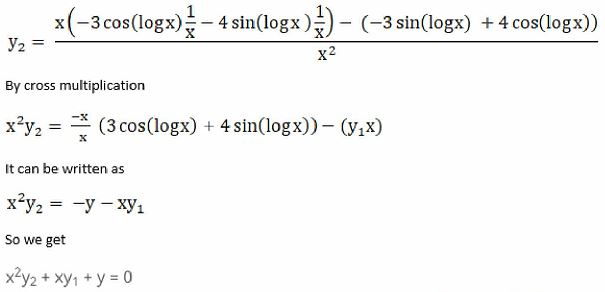Therefore, it is proved.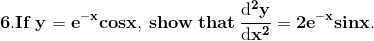Solution: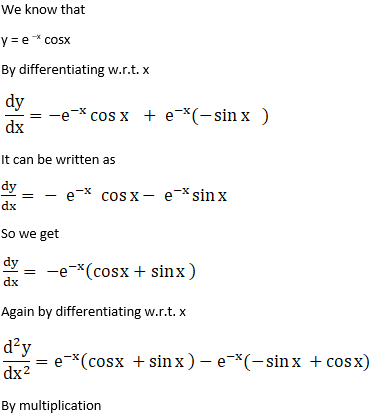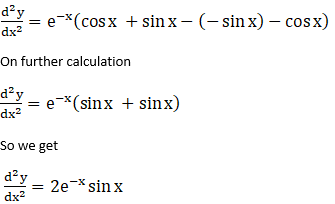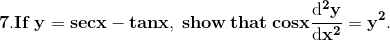Solution: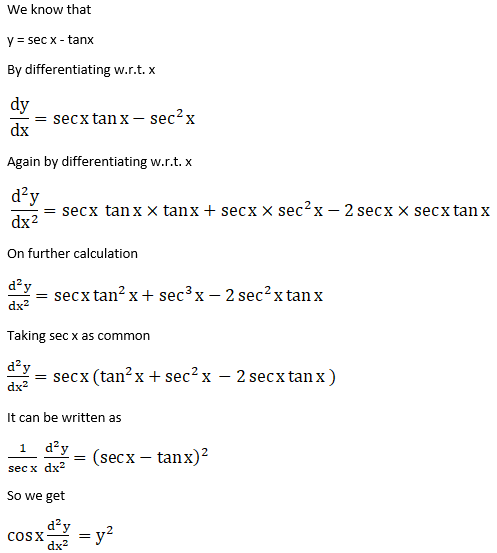Therefore, it is proved.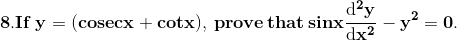Solution: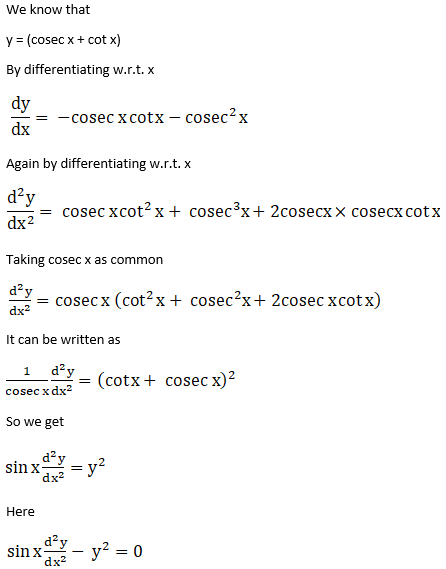Therefore, it is proved.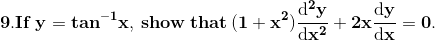Solution: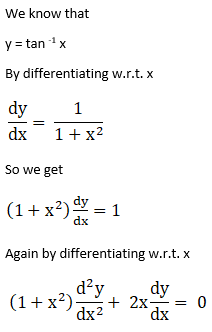Therefore, it is proved.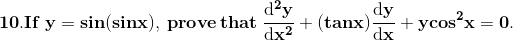Solution: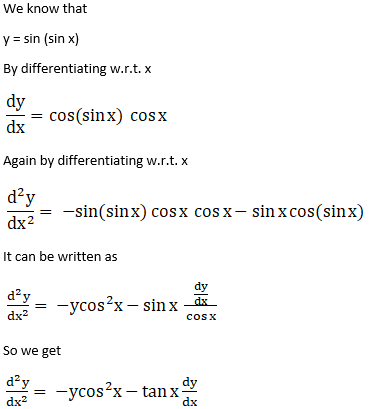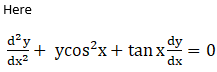11. If y = a cos (log x) + b sin (log x), prove that x2 y2 + xy1 + y = 0

Solution: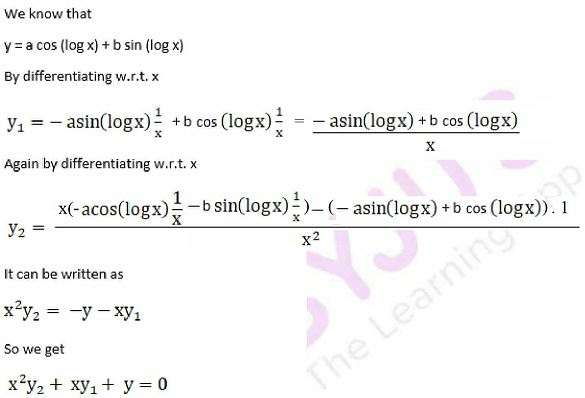Therefore, it is proved.

12. Find the second derivative of e3x sin 4x.

Solution: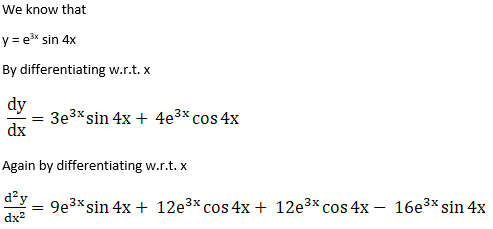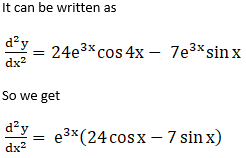13. Find the second derivative of sin 3x cos 5x.

Solution: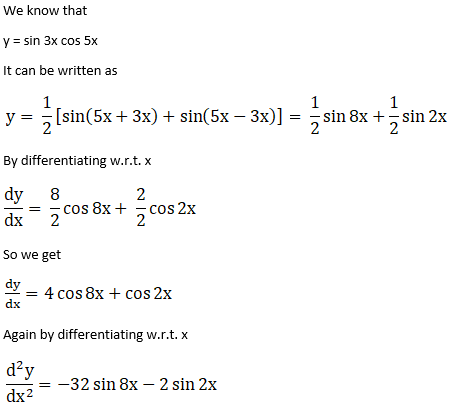Therefore, it is proved.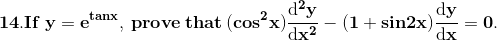Solution: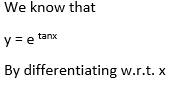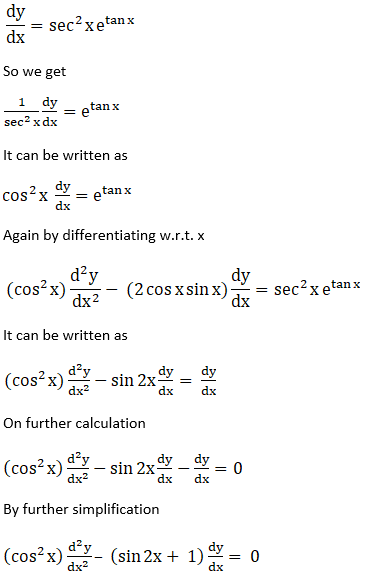Therefore, it is proved.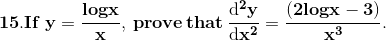Solution: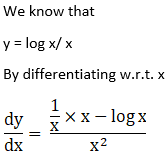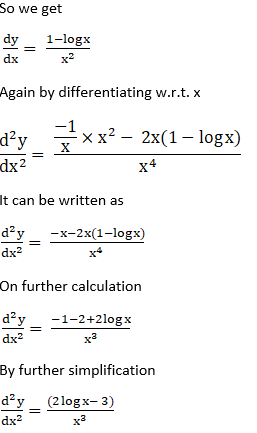Therefore, it is proved.